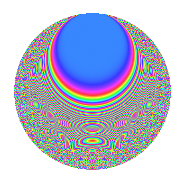Properties

 Label 6017.2.a.eLevel 6017 Weight 2 Character orbit 6017.a Self dual Yes Analytic conductor 48.046 Analytic rank 0 Dimension 119 CM No

Related objects

Newspace parameters

 Level: $$N$$ = $$6017 = 11 \cdot 547$$ Weight: $$k$$ = $$2$$ Character orbit: $$[\chi]$$ = 6017.a (trivial)

Newform invariants

 Self dual: Yes Analytic conductor: $$48.0459868962$$ Analytic rank: $$0$$ Dimension: $$119$$ Fricke sign: $$-1$$ Sato-Tate group: $\mathrm{SU}(2)$

$q$-expansion

The dimension is sufficiently large that we do not compute an algebraic $$q$$-expansion, but we have computed the trace expansion.

 $$\operatorname{Tr}(f)(q) =$$ $$119q$$ $$\mathstrut +\mathstrut 15q^{2}$$ $$\mathstrut +\mathstrut 15q^{3}$$ $$\mathstrut +\mathstrut 133q^{4}$$ $$\mathstrut +\mathstrut 6q^{5}$$ $$\mathstrut +\mathstrut 16q^{6}$$ $$\mathstrut +\mathstrut 72q^{7}$$ $$\mathstrut +\mathstrut 39q^{8}$$ $$\mathstrut +\mathstrut 128q^{9}$$ $$\mathstrut +\mathstrut O(q^{10})$$ $$\operatorname{Tr}(f)(q) =$$ $$119q$$ $$\mathstrut +\mathstrut 15q^{2}$$ $$\mathstrut +\mathstrut 15q^{3}$$ $$\mathstrut +\mathstrut 133q^{4}$$ $$\mathstrut +\mathstrut 6q^{5}$$ $$\mathstrut +\mathstrut 16q^{6}$$ $$\mathstrut +\mathstrut 72q^{7}$$ $$\mathstrut +\mathstrut 39q^{8}$$ $$\mathstrut +\mathstrut 128q^{9}$$ $$\mathstrut +\mathstrut 22q^{10}$$ $$\mathstrut +\mathstrut 119q^{11}$$ $$\mathstrut +\mathstrut 40q^{12}$$ $$\mathstrut +\mathstrut 67q^{13}$$ $$\mathstrut +\mathstrut 3q^{14}$$ $$\mathstrut +\mathstrut 22q^{15}$$ $$\mathstrut +\mathstrut 145q^{16}$$ $$\mathstrut +\mathstrut 57q^{17}$$ $$\mathstrut +\mathstrut 53q^{18}$$ $$\mathstrut +\mathstrut 68q^{19}$$ $$\mathstrut +\mathstrut 25q^{20}$$ $$\mathstrut +\mathstrut 21q^{21}$$ $$\mathstrut +\mathstrut 15q^{22}$$ $$\mathstrut +\mathstrut 21q^{23}$$ $$\mathstrut +\mathstrut 34q^{24}$$ $$\mathstrut +\mathstrut 137q^{25}$$ $$\mathstrut +\mathstrut 10q^{26}$$ $$\mathstrut +\mathstrut 54q^{27}$$ $$\mathstrut +\mathstrut 149q^{28}$$ $$\mathstrut +\mathstrut 46q^{29}$$ $$\mathstrut +\mathstrut 10q^{30}$$ $$\mathstrut +\mathstrut 87q^{31}$$ $$\mathstrut +\mathstrut 58q^{32}$$ $$\mathstrut +\mathstrut 15q^{33}$$ $$\mathstrut +\mathstrut 16q^{34}$$ $$\mathstrut +\mathstrut 40q^{35}$$ $$\mathstrut +\mathstrut 137q^{36}$$ $$\mathstrut +\mathstrut 39q^{37}$$ $$\mathstrut +\mathstrut 27q^{38}$$ $$\mathstrut +\mathstrut 72q^{39}$$ $$\mathstrut +\mathstrut 46q^{40}$$ $$\mathstrut +\mathstrut 50q^{41}$$ $$\mathstrut -\mathstrut 4q^{42}$$ $$\mathstrut +\mathstrut 122q^{43}$$ $$\mathstrut +\mathstrut 133q^{44}$$ $$\mathstrut +\mathstrut 12q^{45}$$ $$\mathstrut +\mathstrut 22q^{46}$$ $$\mathstrut +\mathstrut 92q^{47}$$ $$\mathstrut +\mathstrut 9q^{48}$$ $$\mathstrut +\mathstrut 161q^{49}$$ $$\mathstrut +\mathstrut 2q^{50}$$ $$\mathstrut -\mathstrut 12q^{51}$$ $$\mathstrut +\mathstrut 177q^{52}$$ $$\mathstrut +\mathstrut 12q^{53}$$ $$\mathstrut +\mathstrut 19q^{54}$$ $$\mathstrut +\mathstrut 6q^{55}$$ $$\mathstrut -\mathstrut 16q^{56}$$ $$\mathstrut +\mathstrut 43q^{57}$$ $$\mathstrut +\mathstrut 56q^{58}$$ $$\mathstrut +\mathstrut 39q^{59}$$ $$\mathstrut +\mathstrut 27q^{60}$$ $$\mathstrut +\mathstrut 114q^{61}$$ $$\mathstrut +\mathstrut 66q^{62}$$ $$\mathstrut +\mathstrut 196q^{63}$$ $$\mathstrut +\mathstrut 161q^{64}$$ $$\mathstrut +\mathstrut 7q^{65}$$ $$\mathstrut +\mathstrut 16q^{66}$$ $$\mathstrut +\mathstrut 59q^{67}$$ $$\mathstrut +\mathstrut 139q^{68}$$ $$\mathstrut -\mathstrut 24q^{69}$$ $$\mathstrut +\mathstrut 9q^{70}$$ $$\mathstrut +\mathstrut 11q^{71}$$ $$\mathstrut +\mathstrut 92q^{72}$$ $$\mathstrut +\mathstrut 123q^{73}$$ $$\mathstrut +\mathstrut q^{74}$$ $$\mathstrut +\mathstrut 19q^{75}$$ $$\mathstrut +\mathstrut 92q^{76}$$ $$\mathstrut +\mathstrut 72q^{77}$$ $$\mathstrut -\mathstrut 101q^{78}$$ $$\mathstrut +\mathstrut 78q^{79}$$ $$\mathstrut -\mathstrut 34q^{80}$$ $$\mathstrut +\mathstrut 139q^{81}$$ $$\mathstrut +\mathstrut 73q^{82}$$ $$\mathstrut +\mathstrut 108q^{83}$$ $$\mathstrut -\mathstrut 31q^{84}$$ $$\mathstrut +\mathstrut 30q^{85}$$ $$\mathstrut -\mathstrut 18q^{86}$$ $$\mathstrut +\mathstrut 164q^{87}$$ $$\mathstrut +\mathstrut 39q^{88}$$ $$\mathstrut +\mathstrut 15q^{89}$$ $$\mathstrut -\mathstrut 41q^{90}$$ $$\mathstrut +\mathstrut 60q^{91}$$ $$\mathstrut -\mathstrut 26q^{92}$$ $$\mathstrut -\mathstrut 2q^{93}$$ $$\mathstrut +\mathstrut 45q^{94}$$ $$\mathstrut +\mathstrut 75q^{95}$$ $$\mathstrut +\mathstrut 42q^{96}$$ $$\mathstrut +\mathstrut 73q^{97}$$ $$\mathstrut +\mathstrut 32q^{98}$$ $$\mathstrut +\mathstrut 128q^{99}$$ $$\mathstrut +\mathstrut O(q^{100})$$

Embeddings

For each embedding $$\iota_m$$ of the coefficient field, the values $$\iota_m(a_n)$$ are shown below.

For more information on an embedded modular form you can click on its label.

Label $$a_{2}$$ $$a_{3}$$ $$a_{4}$$ $$a_{5}$$ $$a_{6}$$ $$a_{7}$$ $$a_{8}$$ $$a_{9}$$ $$a_{10}$$
1.1 −2.72929 2.06420 5.44901 −2.31705 −5.63378 0.650725 −9.41333 1.26091 6.32390
1.2 −2.72367 −2.85757 5.41840 −2.70389 7.78308 4.38831 −9.31062 5.16569 7.36452
1.3 −2.68163 0.369101 5.19115 2.09046 −0.989794 1.10498 −8.55750 −2.86376 −5.60585
1.4 −2.67204 2.89488 5.13980 2.14144 −7.73524 4.84141 −8.38968 5.38033 −5.72201
1.5 −2.64305 −0.959171 4.98572 −4.09680 2.53514 −1.46535 −7.89140 −2.07999 10.8280
1.6 −2.64280 −2.08644 4.98439 2.06491 5.51405 1.23299 −7.88714 1.35325 −5.45715
1.7 −2.59415 −0.508113 4.72962 4.04973 1.31812 4.63801 −7.08105 −2.74182 −10.5056
1.8 −2.46359 −1.49738 4.06928 −0.810928 3.68894 4.87400 −5.09785 −0.757845 1.99779
1.9 −2.40399 −2.26995 3.77918 −1.51011 5.45695 −2.08665 −4.27714 2.15269 3.63028
1.10 −2.39877 0.712166 3.75408 0.976168 −1.70832 −3.65167 −4.20764 −2.49282 −2.34160
1.11 −2.37466 0.769590 3.63899 −2.34020 −1.82751 −2.65740 −3.89204 −2.40773 5.55717
1.12 −2.35458 −1.66572 3.54404 1.38814 3.92207 −2.86994 −3.63557 −0.225374 −3.26849
1.13 −2.35115 2.19609 3.52790 0.407257 −5.16332 −2.35563 −3.59233 1.82279 −0.957522
1.14 −2.33754 1.15439 3.46412 −4.35932 −2.69844 3.66625 −3.42244 −1.66738 10.1901
1.15 −2.30862 2.25355 3.32972 2.61387 −5.20258 1.15866 −3.06981 2.07847 −6.03443
1.16 −2.22427 3.08840 2.94737 −2.11023 −6.86944 3.85755 −2.10721 6.53822 4.69372
1.17 −2.14072 −2.03723 2.58269 3.12940 4.36115 −2.52931 −1.24739 1.15032 −6.69918
1.18 −1.98872 0.557360 1.95500 0.637327 −1.10843 2.66044 0.0894893 −2.68935 −1.26746
1.19 −1.97087 1.79688 1.88432 −3.99793 −3.54141 −0.876225 0.227993 0.228774 7.87939
1.20 −1.94884 3.22760 1.79797 1.61357 −6.29006 −0.583291 0.393727 7.41737 −3.14458
See next 80 embeddings (of 119 total)
 $$n$$: e.g. 2-40 or 990-1000 Embeddings: e.g. 1-3 or 1.119 Significant digits: Format: Complex embeddings Normalized embeddings Satake parameters Satake angles

Inner twists

This newform does not have CM; other inner twists have not been computed.

Atkin-Lehner signs

$$p$$ Sign
$$11$$ $$-1$$
$$547$$ $$1$$

Hecke kernels

This newform can be constructed as the intersection of the kernels of the following linear operators acting on $$S_{2}^{\mathrm{new}}(\Gamma_0(6017))$$:

 $$T_{2}^{119} - \cdots$$ $$T_{3}^{119} - \cdots$$